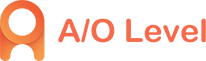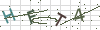• 4008506500
••# A-Level物理考试​知识点：恒定电流

2019-11-12 11:09:57 869人已读

A-Level考试中，物理考试的备考让很多同学都感到头疼，尤其是涉及到一些专业知识点的时候，很多同学都觉得脑子不大够用了，这时候，大家除了要熟记一些公式外，还要多加练习，让自己完全掌握，本文小编就为大家分享一下A-Level物理考试关于恒定电流的知识点，希望能够对大家有所帮助。

恒定电流

1.电流强度：I=q/t{I:电流强度(A)，q:在时间t内通过导体横载面的电量(C)，t:时间(s)｝

2.欧姆定律：I=U/R {I:导体电流强度(A)，U:导体两端电压(V)，R:导体阻值(Ω)｝

3.电阻、电阻定律：R=ρL/S{ρ:电阻率(Ω?m)，L:导体的长度(m)，S:导体横截面积(m2)｝

4.闭合电路欧姆定律：I=E/(r+R)或E=Ir+IR也可以是E=U内+U外

{I:电路中的总电流(A)，E:电源电动势(V)，R:外电路电阻(Ω)，r:电源内阻(Ω)｝5.电功与电功率：W=UIt，P=UI{W:电功(J)，U:电压(V)，I:电流(A)，t:时间(s)，P:电功率(W)｝

6.焦耳定律：Q=I2Rt{Q:电热(J)，I:通过导体的电流(A)，R:导体的电阻值(Ω)，t:通电时间(s)｝

7.纯电阻电路中:由于I=U/R,W=Q，因此W=Q=UIt=I2Rt=U2t/R

8.电源总动率、电源输出功率、电源效率：P总=IE，P出=IU，η=P出/P总

{I:电路总电流(A)，E:电源电动势(V)，U:路端电压(V)，η：电源效率｝

9.电路的串/并联 串联电路(P、U与R成正比) 并联电路(P、I与R成反比)

电阻关系(串同并反) R串=R1+R2+R3+

1/R并=1/R1+1/R2+1/R3+

电流关系 I总=I1=I2=I3 I并=I1+I2+I3+

电压关系 U总=U1+U2+U3+ U总=U1=U2=U3

功率分配 P总=P1+P2+P3+ P总=P1+P2+P3+

10.欧姆表测电阻

(1)电路组成

(2)测量原理

两表笔短接后,调节Ro使电表指针满偏，得

Ig=E/(r+Rg+Ro)

接入被测电阻Rx后通过电表的电流为

Ix=E/(r+Rg+Ro+Rx)=E/(R中+Rx)

由于Ix与Rx对应，因此可指示被测电阻大小

(3)使用方法:机械调零、选择量程、欧姆调零、测量读数{注意挡位(倍率)｝、拨off挡。

(4)注意:测量电阻时，要与原电路断开,选择量程使指针在中央附近,每次换挡要重新短接欧姆调零。

11.伏安法测电阻

电流表内接法： 电流表外接法：

电压表示数：U=UR+UA 电流表示数：I=IR+IV

Rx的测量值=U/I=(UA+UR)/IR=RA+Rx>R真

Rx的测量值=U/I=UR/(IR+IV)=RVRx/(RV+R)

选用电路条件Rx>>RA [或Rx>(RARV)1/2] 选用电路条件Rx<

12.滑动变阻器在电路中的限流接法与分压接法

限流接法

电压调节范围小,电路简单,功耗小 电压调节范围大,电路复杂,功耗较大，便于调节电压的选择条件Rp>Rx 便于调节电压的选择条件Rp

注：

(1)单位换算：1A=103mA=106μA;1kV=103V=106mA;1MΩ=103kΩ=106Ω

(2)各种材料的电阻率都随温度的变化而变化,金属电阻率随温度升高而增大;

(3)串联总电阻大于任何一个分电阻,并联总电阻小于任何一个分电阻;

(4)当电源有内阻时,外电路电阻增大时,总电流减小,路端电压增大;

(5)当外电路电阻等于电源电阻时,电源输出功率最大,此时的输出功率为E2/(2r);

(6)其它相关内容：电阻率与温度的关系半导体及其应用超导及其应用。

以上就是本文关于A-Level物理考试关于恒定电流的知识点介绍了，希望能够对大家有所帮助。如果大家还想了解更多关于中国alevel1月份考试、中国alevel成绩申请国外名校等方面的内容，可以咨询我们的客服或关注网站发布的文章哦~• ### A-level考试备考小心踩中这些雷区4008506500